error: Content is protected !!

# MATHEMATICS: FORM TWO: Topic 9 - TRIGONOMETRY

TOPIC 9: TRIGONOMETRY
Do you want to learn the relationships involving lengths and Angles of right-angled triangle? Here, is where you can learn.
Trigonometry is all about Triangles. In this chapter we are going to deal with Right Angled Triangle. Consider the Right Angled triangle below: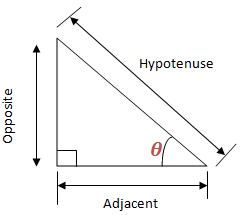The sides are given names according to their properties relating to the Angle .
Opposite side is opposite the Angle
Hypotenuse side is the longest side
Sine, Cosine and Tangent of an Angle using a Right Angled Triangle
Define sine, cosine and tangent of an angle using a right angled triangle
Trigonometry is good at finding the missing side or Angle of a right angled triangle. The special functions, sine, cosine and tangent help us. They are simply one of a triangle divide by another. See similar triangles below: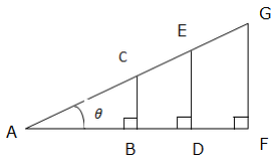The ratios of the corresponding sides are: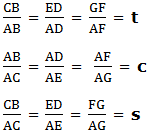Where by tc and s are constant ratios called tangent (t), cosine (c) and sine (s) of Angle respectively.
The right-angled triangle can be used to define trigonometrical ratios as follows:The short form of Tangent is tan, that of sine is sin and that of Cosine is cos.
The simple way to remember the definition of sine, cosine and tangent is the word SOHCAHTOA. This means sine is Opposite (O) over Hypotenuse (H); cosine is Adjacent (A) over Hypotenuse (H); and tangent is Opposite (O) over Adjacent (A). Or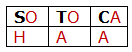Example 1
Given a triangle below, find sine, cosine and Tangent of an angle indicated.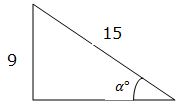Solution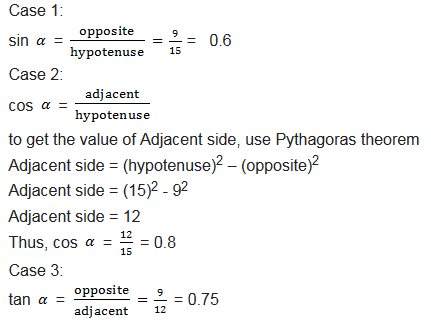Example 2
Given that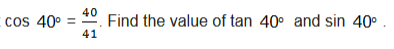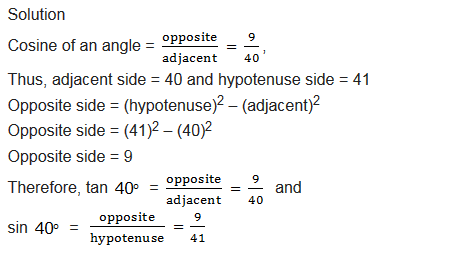Determination of the Sine, Cosine and Tangent of 30°, 45° and 60° without using Mathematical Tables
Determine the sine, cosine and tangent of 30°, 45° and 60° without using mathematical tables
The special Angles we are going to deal with are 300, 450, 600, 900. Let us see how to get the Tangent, Sine and Cosine of each angle as follows:
First, consider an equilateral triangle ABC below, the altitude from C bisects at D.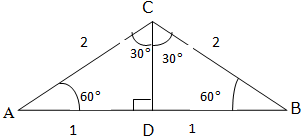AD = BD = 1 (bisection)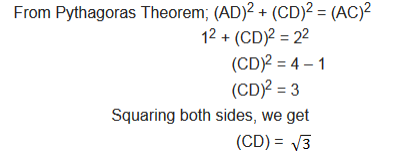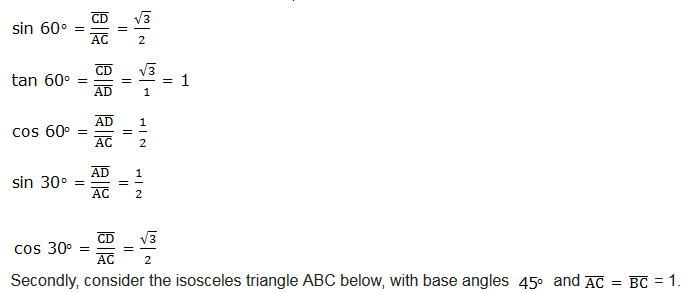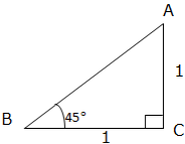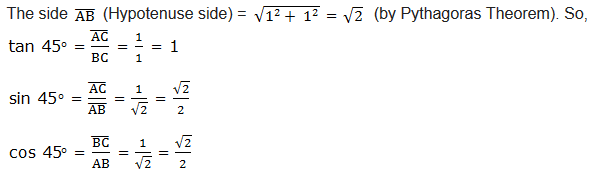The results above can be summarized in table as here below: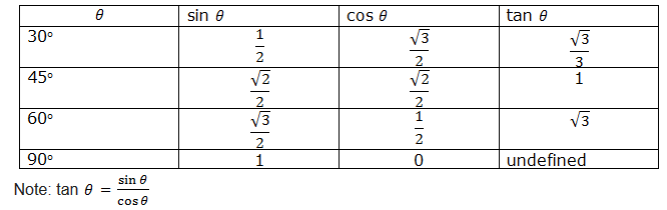Simple Trigonometric Problems Related to Special Angles
Solve simple trigonometric problems related to special angles
Example 3
Find the value of x if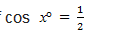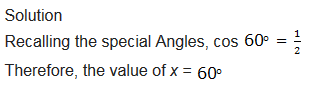The Trigonometric Ratios from Tables
Read the trigonometric ratios from tables
We can find the trigonometrical ratio of any angle by reading it on a trigonometrial table in the same way as we did in reading logarithm of a number on a logarithimic table.
The angle is read from the extreme left hand column and then the corresponding value under the corresponding column of minutes and seconds whenever there is seconds. If we are given angle with zero minute (0’), we read the corresponding value of an angle under the column labeled 0’.
For example; if we are to find the sin560, we have to go to the column extreme to the left. Run your finger down until you meet560, then slide your finger to the exactly same raw to the column labeled 0’. The answer will is 0.8290.
Another example: find cos 78045'. Read the angle780 to the column extreme to the left and then slide your figure to the exactly same angle until you meet the column labeled 45’. The table I’m using has no 45’, so, I have to read the number near to 45’. This number is 42’. The answer of cos78042'is 0.1959. the minutes remained, we are going to read them to the difference columns. Slide your figure to the same column of degree780to the difference column labeled 3’ (minutes remained). The answer is 9. But the instructions says, ‘numbers to the difference columns to be subtracted, not added’. This means we have to subtract 9 (0.0009) from 0.1959. When we subtract we remain with 0.1950. Therefore, cos78045'= 0.1950.
Note that, you can read in the same way the tangent of an angle as we read cosine and sine of an angle. Make sure you read the tables of Natural sine or cosine and or tangent and not otherwise.

Problems involving Trigonometric Ratios from Tables
Solve problems involving trigonometric ratios from tables
Example 4
Use table to find the value of:
1. sin 550
2. cos 34.40
3. tan 60.20
Solution
1. sin 550 = 0.8192
2. To find the value of cos 34.40 , first change34.40 into degrees and minutes. Let us change the decimal part i.e. 0.40 into minutes. 0.4 ×60 minutes = 24 minutes thus, cos 34024' = 0.8251
3. To find the value of tan 60.20, first change60.20 into degrees and minutes. Let us change the decimal part i.e. 0.20 into minutes. 0.2 ×60 minutes = 12 minutes thus tan 60012' = 1.7461
Important note: when finding the inverse of any of the three trigonometric ratios by using table, we search the given ratio on a required table until we find it and then we read the corresponding degree angle. It is the same as finding Ant-logarithm of a number on a table by searching.

Angles of Elevation and Angles of Depression
Demonstrate angles of elevation and angles of depression
Angle of Elevation of an Object as seen by an Observer is the angle between the horizontal and the line from the Object to the Observer’s aye (the line of sight). See the figure below for better understanding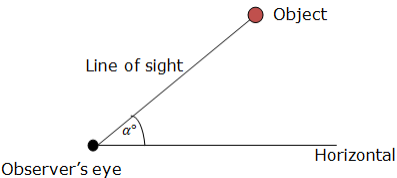The angle of Elevation of the Object from the Observer is α0.
Angle of depression of an Object which is below the level of Observer is the angle between the horizontal and the Observer’s line of sight. To have the angle of depression, an Object must be below the Observer’s level. Consider an illustration below: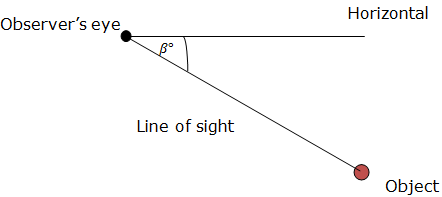The angle of depression of the Object from the Object is β0

Problems involving Angles of Elevation and Angles of Depression
Solve Problems involving angles of elevation and angles of depression
Example 5
From the top of a vertical cliff 40 m high, the angle of a depression of an object that is level with the base of the cliff is350. How far is the Object from the base of the cliff?
Solution
We can represent the given information in diagram as here below: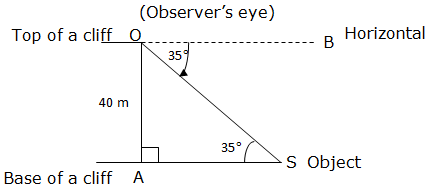Angle of depression = 350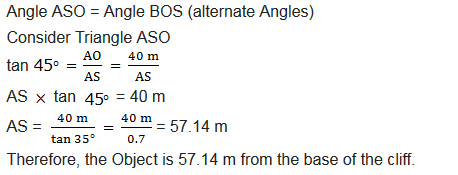Exercise 1
1. Use trigonometric tables to find the following:
1. cos 38.250
2. sin 56.50
3. tan 750
2. Use trigonometrical tables to find the value of x in the following problems.
1. sin x0 = 0.9107
2. tan x0 = 0.4621
3. Find the height of the tower if it casts a shadow of 30 m long when the angle of elevation of the sun is380.
4. The Angle of elevation of the top of a tree of one point from east of it and 56 m away from its base is250. From another point on west of the tree the Angle of elevation of the top is500. Find the distance of the latter point from the base of the tree.
5. A ladder of a length 15m leans against a wall and make an angle of300with a wall. How far up the wall does it reach?